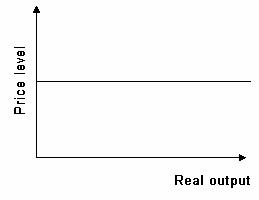### CFA Practice Question

There are 539 practice questions for this study session.

### CFA Practice Question

Refer to the graph below. The short-run curve in the graph is called a(n) ______.A. aggregate supply curve
B. potential output curve
C. firm's supply curve

The aggregate supply curve is horizontal in the short run because most U.S. markets are quantity-adjusting markets.

User Comment
Gina the AS has 3 ranges 1) horizontal range (like in the quiz), where the price level remains constant as domestic output varies 2) upsloping range, where price level and real domestic output can vary 3) vertical range, where real domestic output is constant at FE level and only price level can vary